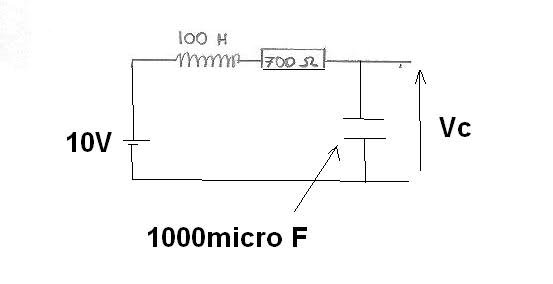# How long does it take a Capacitor to charge to 80% of its final value?

#### MarkH748

1. The problem statement, all variables and given/known data

I have a a circuit with an inductor, a resistor and a capacitor in series. I have analyzed the circuit and gotten an equation for the voltage across the capacitor to be.2. Relevant equations

Vc(t) = 10 + (20/3)e^-5t - (50/3)e^-2t

3. The attempt at a solution

I assume its something to do with time constants but there isnt any that give me 80% is there? Also my electronics isnt that great so I don't know how to deal with the inductor.

Any help would be greatly appreciated.

Mark.
1. The problem statement, all variables and given/known data

2. Relevant equations

3. The attempt at a solution

#### ehild

Homework Helper
What is the limit of Vc when tends to infinity? You need to determine the time t when Vc is 80 percent of this value. You can solve the equation only numerically, but the result will be close to 0.95 s.

ehild

#### MarkH748

I'm still unsure. Do I say @ t = infinity, Vc = 10 - 700I ? I don't have a vlue for I though. Do I need one? I'd also be interested to know how you estimated 0.95s. Could I write in an exam, "By observation, t = 0.95s" ? or would that be meaningless?

#### ehild

Homework Helper
Vc(t) = 10 + (20/3)e^-5t - (50/3)e^-2t

This is the equation which gives the voltage of the capacitor as function of time. I do not know if you have learnt about differential equations or not, but it is the solution of the circuit equation - a second order differential equation for the charge on the capacitor.

Now you have no other task, find the limit of the Vc(t) function when the time goes to infinity. What happens then with the exponential functions?

You can solve an equation numerically by trying different values, or with some kind of iteration. You can also solve it graphically: Plot it out.

ehild

#### MarkH748

I think I understand now. When t -> infinity, Vc = 10 V (this is correct?). So I need to find a value for t for when Vc is 8 volts using one of the methods you mentioned?

edit: this gives me t = 3.6188.

Last edited:

#### ehild

Homework Helper
Yes, you can find t when Vc is 8 V. But your t is too big. Try to plug in. How did you get it?

ehild

#### MarkH748

Ah, I put V equal to 2 volts by accident. Putting it equal to 8 gives me t = 0.9047 s. Thanks for your help.

Homework Helper
You are welcome.

ehild

### The Physics Forums Way

We Value Quality
• Topics based on mainstream science
• Proper English grammar and spelling
We Value Civility
• Positive and compassionate attitudes
• Patience while debating
We Value Productivity
• Disciplined to remain on-topic
• Recognition of own weaknesses
• Solo and co-op problem solving